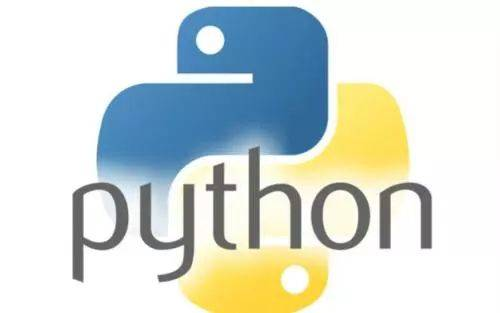• 原标题：Python字符串加密解密方法总结 编程中经常会对字符串做加密解密处理，特别是涉及到隐私的字符串，如密码等的时候，就需要加密，自己总结了一下，大致有三种：，win32com.client自己写加密解密算法，当然最...
原标题：Python字符串加密解密方法总结编程中经常会对字符串做加密解密处理，特别是涉及到隐私的字符串，如密码等的时候，就需要加密，自己总结了一下，大致有三种：，win32com.client和自己写加密解密算法，当然最安全的就是自己写加密解密算法了。1. 最简单的方法是用:imports1 = .encodestring('hello world')s2 = .decodestring(s1)print s1,s2# aGVsbG8gd29ybGQ=\n# hello world注: 这是最简单的方法了，但是不够保险，因为如果别人拿到你的密文，也可以自己解密来得到明文;不过可以把密文字符串进行处理，如字母转换成数字或是特殊字符等，自己解密的时候在替换回去在进行.decodestring，这样要安全很多。2. 第二种方法是使用win32com.clientimport win32com.clientdef encrypt(key,content): # key:密钥,content:明文EncryptedData = win32com.client.Dispatch('CAPICOM.EncryptedData')EncryptedData.Algorithm.KeyLength = 5EncryptedData.Algorithm.Name = 2EncryptedData.SetSecret(key)EncryptedData.Content = contentreturn EncryptedData.Encrypt()def decrypt(key,content): # key:密钥,content:密文EncryptedData = win32com.client.Dispatch('CAPICOM.EncryptedData')EncryptedData.Algorithm.KeyLength = 5EncryptedData.Algorithm.Name = 2EncryptedData.SetSecret(key)EncryptedData.Decrypt(content)str = EncryptedData.Contentreturn strs1 = encrypt('lovebread', 'hello world')s2 = decrypt('lovebread', s1)print s1,s2# MGEGCSsGAQQBgjdYA6BUMFIGCisGAQQBgjdYAwGgRDBCAgMCAAECAmYBAgFABAgq# GpllWj9cswQQh/fnBUZ6ijwKDTH9DLZmBgQYmfaZ3VFyS/lq391oDtjlcRFGnXpx# lG7o# hello world注: 这种方法也很方便，而且可以设置自己的密钥，比第一种方法更加安全，如果对安全级别要求不太高的话这种方法是加密解密的首选之策!3. 还有就是自己写加密解密算法，比如:def encrypt(key, s):b = bytearray(str(s).encode(gbk))n = len(b) # 求出 b 的字节数c = bytearray(n*2)j = 0for i in range(0, n):b1 = b[i]b2 = b1 ^ key # b1 = b2^ keyc1 = b2 % 16c2 = b2 // 16 # b2 = c2*16 + c1c1 = c1 + 65c2 = c2 + 65 # c1,c2都是0~15之间的数,加上65就变成了A-P 的字符的编码c[j] = c1c[j+1] = c2j = j+2return c.decode(gbk)def decrypt(key, s):c = bytearray(str(s).encode(gbk))n = len(c) # 计算 b 的字节数if n % 2 != 0 :returnn = n // 2b = bytearray(n)j = 0for i in range(0, n):c1 = c[j]c2 = c[j+1]j = j+2c1 = c1 - 65c2 = c2 - 65b2 = c2*16 + c1b1 = b2^ keyb[i]= b1try:return b.decode(gbk)except:return failedkey = 15s1 = encrypt(key, 'hello world')s2 = decrypt(key, s1)print s1,'\n',s2# HGKGDGDGAGPCIHAGNHDGLG# hello world注: 这是网上抄来的一个简单的例子，大家可以自定义自己算法进行加密解密;还有许许多多复杂的加密算法，大家可以自行查阅密码学的相关算法。4.对于python来说，也可以把python源码文件编译成pyc二进制格式的文件，这样别人就看不到你的源码，也算是一种加密方法吧，方法如下：执行命令python -m py_compile create_slave.py 可以直接生成一个create_slave.pyc文件，然后可以用create_slave.pyc来替换create_slave.py作为脚本来执行。返回搜狐，查看更多责任编辑：
展开全文• 不过可以把密文字符串进行处理， 如字母转换成数字或是特殊字符等， 自己解密的时候在替换回去在 进行 base64.decodestring ，这样要安全很多。 13. 14. 15. 16. 17.2. 第二种方法是使用 win32...
1.1.最简单的方法是用base64:2.3.import base644.5.s1 = base64.encodestring('hello world')6.s2 = base64.decodestring(s1)7.print s1,s28.9.# aGVsbG8gd29ybGQ=\n10.# hello world11.12.注:这是最简单的方法了，但是不够保险，因为如果别人拿到你的密文，也可以自己解密来得到明文；不过可以把密文字符串进行处理，如字母转换成数字或是特殊字符等，自己解密的时候在替换回去在进行base64.decodestring，这样要安全很多。13.14.15.16.17.2.第二种方法是使用win32com.client18.19.import win32com.client20.def encrypt(key,content):# key:密钥,content:明文21.    EncryptedData = win32com.client.Dispatch('CAPICOM.EncryptedData')22.    EncryptedData.Algorithm.KeyLength = 523.    EncryptedData.Algorithm.Name = 224.    EncryptedData.SetSecret(key)25.    EncryptedData.Content = content26.    return EncryptedData.Encrypt()27.28.def decrypt(key,content):# key:密钥,content:密文29.    EncryptedData = win32com.client.Dispatch('CAPICOM.EncryptedData')30.    EncryptedData.Algorithm.KeyLength = 531.    EncryptedData.Algorithm.Name = 232.    EncryptedData.SetSecret(key)33.    EncryptedData.Decrypt(content)34.    str = EncryptedData.Content
展开全文• 进行加密解密 设计应用程序时，为了防止一些敏感信息的泄露，通常需要对这些信息进行加密。 以用户的登录密码为例，如果密码以明文的形式存储在数据表中，很容易就会被人发现；相反，如果密码以密文的形式储存...

符串进行加密与解密      设计应用程序时，为了防止一些敏感信息的泄露，通常需要对这些信息进行加密。     以用户的登录密码为例，如果密码以明文的形式存储在数据表中，很容易就会被人发现；相反，如果密码以密文的形式储存，即使别人从数据表中发现了密码，也是加密之后的密码，根本不能使用。通过对密码进行加密，能够极大地提高系统的保密性。加密与解密:    加密的方法一经公开,就不成其为密. 所以你要你的加密方法还没有被破解,就可以使用.   加密就象是变戏法, 戏法人人会变,巧妙各有不同.加密字符串的思路:      s1 = jiami ( s )        s 称为原文,   s1 称为密文    如果从 s1 存在一个函数 ffjiami( s1 ) 求出 s , 称 jiami 是可逆变换. 否则称为不可逆变换.        本文介绍的是可逆变换加密方法的例子.(1)   将字符串s 变为 bytearray 数组        b = byteaaray( s.encode( "gbk"))(2)    将 b 经过某种变换 成为另一个 字节数组 c      关键是 这种变换应该是可逆的, 并且保证 c 能够通过 下面的第(3) 变为一个字符串.(3) 将 c 转换成普通字符串      s1 = c.decode( "gbk")( 4 ) 解密过程是上述过程的逆过程#coding=gbk # 字符串加密初探 # 入口 : s 要加密的串 #        key 你的密钥 一个字节 1~255之间的整数 # 返回: 加密后的串 def jiaMi( s , key ):     b = bytearray( str(s).encode("gbk") )     n = len(b) # 求出 b 的字节数     c = bytearray( n*2 )     j = 0     for i in range( 0, n ):         b1 = b[i]         b2 = b1 ^ key     # b1 = b2^ key         c1 = b2 % 16         c2 = b2 // 16     # b2= c2*16 + c1         c1 = c1 + 65         c2 = c2 + 65      # 由于c1,c2都是 0~15之间的数,                           # 加上65就变成了A-P 的字符的编码         c[j]    = c1         c[j+1] = c2         j = j+2     return c.decode("gbk")def ffjiaMi( s, key ):     c = bytearray( str(s).encode("gbk") )     n = len(c) # 求出 b 的字节数     if n % 2 != 0 :         return ""     n = n // 2     b = bytearray( n )     j = 0     for i in range( 0, n ):         c1 = c[j]          c2 = c[j+1]          j   = j+2         c1 = c1 - 65         c2 = c2 - 65         b2 = c2*16 + c1         b1 = b2^ key         b[i]= b1     try:          return b.decode("gbk")     except:       return "解密失败"key = 15s   = "my dear black hole , haha! " s1 = jiaMi( s, key ) s2 = ffjiaMi( s1,key ) print( "原文=", s) print( "密文=", s1) print( "解密:") print( s2 )
-- 结果 ---原文= my dear black hole , haha! 密文= CGGHPCLGKGOGNHPCNGDGOGMGEGPCHGAGDGKGDCPCHGOGHGOGOC解密: my dear black hole , haha!

转载于:https://blog.51cto.com/linuxshow/784239
展开全文• # c1,c2都是0~15之间的数,加上65就变成了A-P 的字符的编码 c [ j ] = c1 c [ j + 1 ] = c2 j = j + 2 return c . decode ( gbk ) 　 def decrypt ( key , s ) : c = bytearray ( str ( s...

def encrypt(key, s):
b = bytearray(str(s).encode(gbk))
n = len(b) # 求出 b 的字节数
c = bytearray(n*2)
j = 0
for i in range(0, n):
b1 = b[i]
b2 = b1 ^ key # b1 = b2^ key
c1 = b2 % 16
c2 = b2 // 16 # b2 = c2*16 + c1
c1 = c1 + 65
c2 = c2 + 65 # c1,c2都是0~15之间的数,加上65就变成了A-P 的字符的编码
c[j] = c1
c[j+1] = c2
j = j+2
return c.decode(gbk)

def decrypt(key, s):
c = bytearray(str(s).encode(gbk))
n = len(c) # 计算 b 的字节数
if n % 2 != 0 :
return
n = n // 2
b = bytearray(n)
j = 0
for i in range(0, n):
c1 = c[j]
c2 = c[j+1]
j = j+2
c1 = c1 - 65
c2 = c2 - 65
b2 = c2*16 + c1
b1 = b2^ key
b[i]= b1
try:
return b.decode(gbk)
except:
return failed

key = 15
s1 = encrypt(key, 'hello world')
s2 = decrypt(key, s1)
print s1,'\n',s2
# HGKGDGDGAGPCIHAGNHDGLG
# hello world



展开全文• 编程中经常会对字符串加密解密处理，特别是涉及到隐私的字符串，如密码等的时候，就需要加密，自己总结了一下，大致有三种：base64，win32com.client自己写加密解密算法，当然最安全的就是自己写加密解密算法了...
• 在生产中会遇到很多情况是需要加密字符串的（如访问或存储密码）这些需求造就了需要字符串加密，以及反解密的问题，推荐两种方法来实现，下附代码： #!/usr/bin/env python3 # -*- coding: utf-8 -*- def ...
• Note: 这是最简单的方法了，但是不够保险，因为如果别人拿到你的密文，也可以自己解密来得到明文 2. 第二种方法是使用win32com.client 复制代码 代码如下:import win32com.clientdef encrypt(key,content): # key:...
•编程语言
• 字符串加密解密题目描述解题思路python代码实现 题目描述 输入两行字符串，第一行为需要加密的字符串，第二行为需要解密的字符串，按照加密解密规则输出两行，第一行为加密后的字符串，第二行为解密后的字符串。加密...
• 本文实例讲述了Python实现对字符串加密解密方法。分享给大家供大家参考，具体如下：需求是是要将密码存在数据库里，所以要加密解密是可逆的，在数据库里不要有特殊字符，防止数据库备份恢复中出错。安装PyCrypto...
• python字符串佛曰加密 #加密 #维吉尼亚密码 str1 = "abcdefghi,.;:jklmnop!qrstuvwxyz12 346578ABCDEFGHIJKLMNOPQRSTUVWXYZ" str2 = "klmnopqr12s3t,A:5;B.X6DE8!FGH 7IJKL4MNOPQRSTUVWXYZuvwxyzabcdefghij" str3 = ...
• 主要介绍了Python实现对字符串加密解密方法,结合实例形式分析了Python使用PyCrypto模块进行DES加密解密的相关操作技巧,需要的朋友可以参考下
• 本文实例讲述了Python实现对字符串加密解密方法。分享给大家供大家参考，具体如下：需求是是要将密码存在数据库里，所以要加密解密是可逆的，在数据库里不要有特殊字符，防止数据库备份恢复中出错。安装PyCrypto...
• 本文实例为大家分享了python实现字符串加密成纯数字的具体代码，供大家参考，具体内容如下说明:该加密算法仅仅是做一个简单的加密,安全性就不谈了,哈哈.算法流程:1.字符串以utf8编码成字节数组2.把每一个字节转换成...
• 1、 安装教程 安装步骤教程 ... pip3 install wheel ...pycrypto，pycrytodomecrypto是一个东西，crypto在python上面的名字是pycrypto它是一个第三方库，但是已经停止更新三年了，所以不建议安装这个库；...
• 本文实例为大家分享了python实现字符串加密成纯数字的具体代码，供大家参考，具体内容如下说明:该加密算法仅仅是做一个简单的加密,安全性就不谈了,哈哈.算法流程:1.字符串以utf8编码成字节数组2.把每一个字节转换成...
• 算法描述不管输入字符串多长，把该字符串填到一个刚好合适的N*N的正方形的格子中，然后以对角线为对称线，对格子里的字母进行交换，然后对折进行交换，列如，输入abcd则填入2x2的方格中：abcd先对角线交换，变为：...
• import base64 import matplotlib.pyplot as plt # plt 用于显示图片 import numpy as np from PIL import Image # f = open('mima.txt',"r"); # linedata = f.read() # f.close() linedata='MG4...
• 本文实例为大家分享了python实现移位加密和解密的具体代码，供大家参考，具体内容如下 代码很简单，就不多做解释啦。主要思路是将字符串转为Ascii码，将大小写字母分别移位密钥表示的位数，然后转回字符串。需要......

# python字符串加密和解密python 订阅